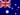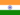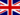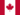All Modules View all topics Close topics
Module 1: Équations et inéquations

# Résoudre des équations et des expressions de simplifier

• Notes d'étude
• Révisions du sujetK C. 6 0 Wrong equation. Please be more careful so that no one really gets confused.Barry L. 3 2 Now can we have a video that solves the equation that was on the previous page... 12(3b-b/4a)=36 ?Mystear P. 5 2 How can we put the 4a below 12 a? My teacher told us that we can't do that because 12a x 2b/4a isn't the same as 12a/4a x 2b. And now I'm confused. Can anyone explain?Mireshkumar S. 2 1 Wrong equation to solve!Hanna M. 0 0 I think you might of typed the equation for us to solve wrong as you gave us 12(3b-b/ 4a)=36 to solve, however you solved 12a(3b-b/ 4a)=36. please can you correct this and ensure no further mishaps. thanks.Chris C. 0 1 This is great I learn the equation and the process to simplify.Chris C. 1 0 Do you always pull out the 4aHabiba A. 0 0 its interesting. I want to do thisHabiba A. 2 0 (5x - 2x)/3 = 6Mya M. 1 1 In one of algebraic the question is 12(3b-b/ 4a)=36 However the solution suggests that coefficient is 12a and not 12 which would've entailed a simplistic outcome for students attempting to achieve the solution on their own. What be the difference in the outcome if the problem stayed the same and didn't have a 12a coefficient as it does in the solution?